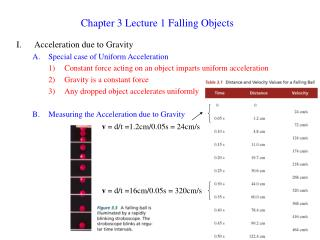Download PresentationChapter 3 Lecture 1 Falling Objects

# Chapter 3 Lecture 1 Falling Objects - PowerPoint PPT Presentation

Chapter 3 Lecture 1 Falling Objects. Acceleration due to Gravity Special case of Uniform Acceleration Constant force acting on an object imparts uniform acceleration Gravity is a constant force Any dropped object accelerates uniformly Measuring the Acceleration due to Gravity.I am the owner, or an agent authorized to act on behalf of the owner, of the copyrighted work described.
Download Presentation## Chapter 3 Lecture 1 Falling Objects

Download Policy: Content on the Website is provided to you AS IS for your information and personal use and may not be sold / licensed / shared on other websites without getting consent from its author.While downloading, if for some reason you are not able to download a presentation, the publisher may have deleted the file from their server.

- - - - - - - - - - - - - - - - - - - - - - - - - - E N D - - - - - - - - - - - - - - - - - - - - - - - - - -
Presentation Transcript
1. Chapter 3 Lecture 1 Falling Objects • Acceleration due to Gravity • Special case of Uniform Acceleration • Constant force acting on an object imparts uniform acceleration • Gravity is a constant force • Any dropped object accelerates uniformly • Measuring the Acceleration due to Gravity v = d/t =1.2cm/0.05s = 24cm/s v = d/t =16cm/0.05s = 320cm/s

2. Dy • Compute average velocity for each time period • Plot velocity versus time • Remember • Compute acceleration due to gravity: g = 9.8 m/s2 • Air Resistance • Which hits first, brick or a feather? • g is constant for all things; weight of the object doesn’t matter • Shape of a feather and its light weight do cause it to interact with the air strongly = air resistance • Large surface area • Small weight • In a vacuum (no air) all things fall at the same rate (on Earth) Dx

3. v = vo + at d = vot + ½ at2 • Tracking Falling Objects • Ball dropped from a building • Find velocity and distance at 0.5-2 s • Plot distance vs. time

4. Throwing a ball straight down • Now we have an initial velocity v0 • Acceleration due to gravity is still g = 9.8 m/s2 • Ball is thrown down at 20 m/s. What are the velocities and distances at 1 and 2 seconds? • Throwing a ball straight up • Direction of velocity and acceleration are very important • What is the acceleration at the top of the trajectory? What is the velocity? - a - a + v - v

5. 3) Example: sample exercise 3.2